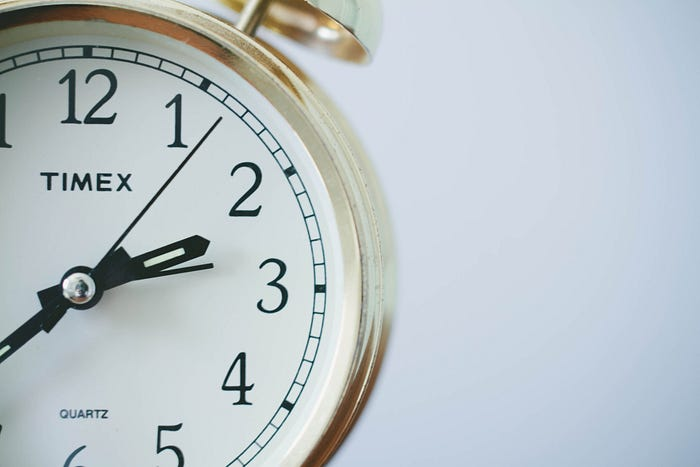# How to Convert the Number of Seconds to a Time String in the hh:mm:ss Format with JavaScript?Sometimes, we may want to convert the number of seconds elapsed to a time string in the hh:mm:ss format in our JavaScript app.

In this article, we’ll look at how to convert the number of seconds to a time string in hh:mm:ss format.

### Create Our Own Function

We can create our own function to convert the number of seconds elapsed to a time string in hh:mm:ss format.

For instance, we can write:

``````const toHHMMSS = (numSecs) => {
let secNum = parseInt(numSecs, 10);
let hours = Math.floor(secNum / 3600)
.toString()
let minutes = Math.floor((secNum - hours * 3600) / 60)
.toString()
let seconds =
secNum - hours * 3600 - (minutes * 60).toString().padStart(2, "0");
return `\${hours}:\${minutes}:\${seconds}`;
};
console.log(toHHMMSS(1234));
``````

In the `toHHMMSS` function, we parse the `numSecs` parameter into a number.

Then we compute the hours by dividing `secNum` by 3600.

Then we round it down to the nearest integer with `Math.floor` .

Then we call `toString` on it to convert it into a string.

And then we call `padStart` to pad the number string to 2 digits with a leading zero if necessary.

To compute the `minutes` , we subtract `secNum` by the `hours` multiplied by 3600 and divide the whole thing by 60.

We get the minutes after subtracting the hours.

And we round the number down to the nearest integer with `Math.floor` .

Then we pad it into a 2 digit string with `padStart` also.

Next, we compute the `seconds` by subtracting the `hours` and `minutes` converted to seconds from `secNum` .

Then we convert it to a string and call `padStart` on it to pad the string to 2 digits with a leading zero if necessary.

And finally, we return the `hours` , `minutes` , and `seconds` in a string.

Therefore, the console log should log:

``````'00:20:34'
``````

### Date.prototype.toISOString

We can call `toISOString` on the JavaScript date object to get a date string with the hours, minutes, and seconds.

To get the elapsed time, we just create a date with timestamp 0, then call `setSeconds` to set the timestamp to the elapsed seconds.

And then we can all `toISOString` to get the date string and extract the hours, minutes, and seconds part of the returned date string.

For instance, we can write:

``````const date = new Date(0);
date.setSeconds(1234);
const timeString = date.toISOString().substr(11, 8);
console.log(timeString);
``````

We create a `Date` instance with timestamp 0.

Then we call `setSeconds` to set the seconds of the time.

And then we call `toISOString` to get a date string.

Then we call `substr` to extract the hours, minutes, and seconds substring.

Therefore, `timeString` is:

``````'00:20:34'
``````

### Conclusion

We can use math, date, and string methods to get the elapsed seconds into hh:mm:ss format easily with JavaScript.